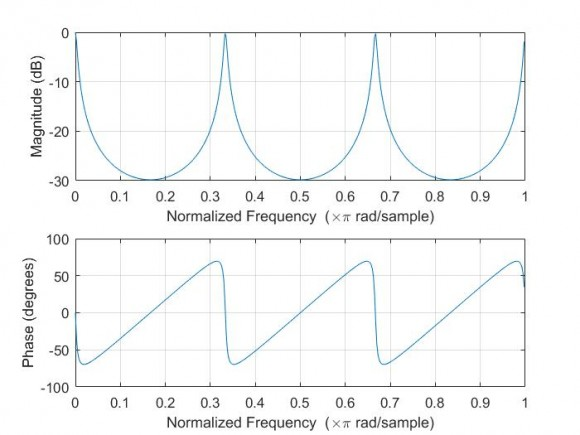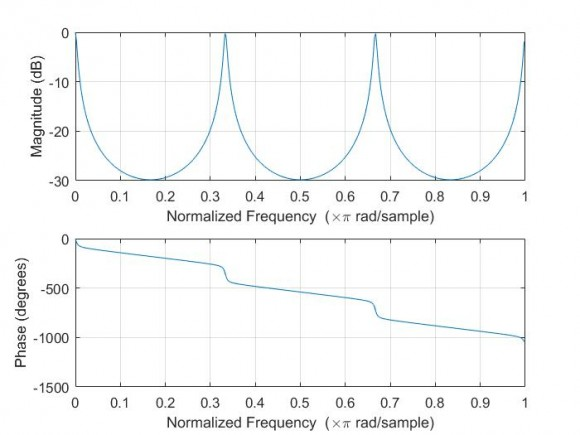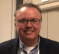## Phase in a filterStarted by 4 years ago11 replieslatest reply 4 years ago176 views

Hello

I am a bit confused with the phase response of filters... I think I do not yet understand completly the topic... I'm playing with a very basic filter... a recursive peaking comb... and when I combine the filter with a delay (two systems in series with the delay either before or after) I get a very weird phase response...

The original filter is $$H[z] = \frac{(1-a)}{(1-az^{-N})}$$ while the filter delayed (again combination of the original pluse a delay) is $$H[z] = \frac{(1-a)z^{-N}}{(1-az^{-N})}$$

For the both I get the same magnitude response as expected... but when plotting with matlab the first increases and decreases with phase jumps in the peaks while the second one is giving me kind of decreasing phase with pahse jumps in the peaks...  you can see in the following plots, the original;The delayed;Could somebody add some light to the physical meaning of this difference and why it happens only delaying the response of the filter?

Thank you

[ - ]To see what's happening, separate the filter into 2 parts.

Plot the phase of z^-N.  You are just adding that phase to the original.

[ - ]The gain responses in series filters are accumulated multiplicatively point-wise by frequency.  The phase responses in series filters are accumulated additively point-wise by frequency.  If the first filter has a gain of 2 and 90deg of lag at 100Hz and the second has a of 0.5 and 5deg of phase lag at 100Hz, the net result will be a gain of 1 and 95deg of phase lag at 100Hz.  If you plot the gain and phase response of your pure delay, you will see unity gain and a monotonically decreasing phase in the form of a straight line.  Your phase result may be more clear, then.  It is simply the point-wise addition of your original comb filter phase and the delay phase.

[ - ]can you show how you enter matlab to get mag/phase please

[ - ]Hi Kaz,

mag: plot(20*log10(abs(H)));

phase:  plot(phase(H));

plot(angle(H)); if you wanted wrapped

[ - ]I can see your work is correct and the replies given here are useful.

To explain it there are two perspectives:

1) complicated one say you got two systems:

assume a = .5;

freqz(1-a,[1 0 0 0 0 0 -a])

freqz([0 0 0 0 0 1-a],[1 0 0 0 0 0 -a])

2) simple case of delay.

for a given input your output is exactly same stream apart from constant delay of 5 samples delay.

grpdelay(1-a,[1 0 0 0 0 0 -a])

grpdelay([0 0 0 0 0 1-a],[1 0 0 0 0 0 -a])

[ - ]total_delay[n] = org_delay[n] + const_delay[n]

org_delay[.] = a 3 cycle sawtooth with pp of +/-60 degree.

const_delay[.] = half cycle sawtooth starting with 0 and ending at -1080(3*360).

if you do the above math the plot is going to be.[ - ]Hi All, thanks for the quick reply... and going a bit depper in the question... and looking for a physical meaning of that phase...

a) I understand that for the combined filter(delay and IIR with normalized gain) the "phase shift" the it introduces to a single tone depends in the frequency of the tone, (according to the plot for 0.9 normalized tone it would be a bit less than 1000 deg), and that this value increases with ferquency due to the delay which has a stepest slope than the IIR. Is this right?

b) Looking only the IIR it seems that the phase shift introduced by the filter is sometimes positive, and some times negative... I know that linear phase shif filters are FIRs and not IIRs... but is it normal the IIR has a positive slope instead of a negative one, and some times adds a positve shift and others a negative?

c) Looking in detail the resonances... there we find "phase jumps"... In the plots I can see these are arond 120deg (+/-60deg)... should this be 180 instead of 120?

d) A bit more of fun with phase delay and group delay, when I computed them and plot it in the following picture (top only filter, middle only delay, bottom combination)I can understand that phase delay for the pure delay is the time it takes any tone to "cross" the filter, which is the same for any frequency... but then in the other plots... this is variable... does this make sense looking it from a physics point of view? should any signal take the same time independent of the frequency to "cross" the filter? why we don't see this behaviour then in the IIR (I understand again the combined one is the combination of IIR and delay)... and I'm lost with the group delay...

Thanks a lot

[ - ]I'm on my phone, so I can't give so great of a response, but here it goes...  Filters are realized by storing and releasing energy in controlled ways.  That means that a filter in steady state operation can do many interesting things.  That same filter - when fed an impulse with uncharged initial conditions - has no stored energy and, as such, responds quite unremarkably.  ...or unexpectedly.  If you subjected your comb filter to a slow sine sweep on the bench after letting its storage elements load up, you would indeed see the relative phase from output-to-input lead.  ...but energy is not being created.  It is only being maintained.  When you, then, further consider that FIR filters can only operate on inputs and IIR filters can operate on both inputs and past outputs, the possible outcomes of relative phase become more clear.
[ - ]Hi Rockymarx,

phase delay is not how much time it takes to "cross the filter". It is how many periods of the sinewave it takes to do so, that's why it's different for each frequency. You are confusing it with a group delay - which is exactly the time it takes for each frequency to go through the system and it is constant for a constant delay line.

The group delay is a negated derivative of the phase, so linearly decaying phase response means constant positive time delay.

Best regards,
AXP

[ - ]Hi AXP,

I think Rockymarx meant constant delay when he mentioned phase delay.

obviously these terms constant delay,phase delay,group delay are confusing

[ - ]